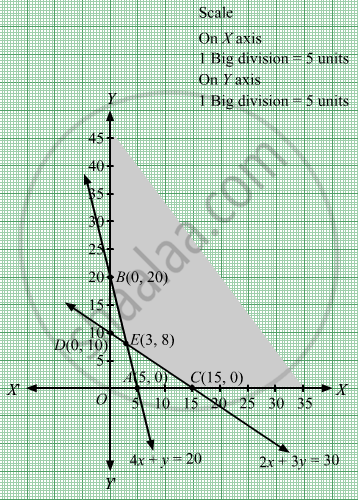# Minimize Z = 18x + 10y Subject to 4 X + Y ≥ 20 2 X + 3 Y ≥ 30 X , Y ≥ 0 - Mathematics

Minimize Z = 18x + 10y
Subject to

$4x + y \geq 20$
$2x + 3y \geq 30$
$x, y \geq 0$

#### Solution

First, we will convert the given inequations into equations, we obtain the following equations:
4x y = 20, 2x +3y = 30, x = 0 and y = 0

Region represented by 4x y ≥ 20 :
The line 4x y = 20  meets the coordinate axes at A(5, 0) and B(0, 20) respectively. By joining these points we obtain the line 4x y = 20.
Clearly (0,0) does not satisfies the inequation 4x y ≥ 20. So,the region in xy plane which does not contain the origin represents the solution set of the inequation 4x y≥ 20.

Region represented by 2x +3y ≥ 30:
The line 2x +3y = 30 meets the coordinate axes at C(15,0) and D(0, 10) respectively. By joining these points we obtain the line
2x +3y = 30.Clearly (0,0) does not satisfies the inequation 2x +3y ≥ 30. So,the region which does not contain the origin represents the solution set of the inequation 2x+3y ≥ 30.

Region represented by x ≥ 0 and y ≥ 0:
Since, every point in the first quadrant satisfies these inequations. So, the first quadrant is the region represented by the inequations x ≥ 0, and ≥ 0.
The feasible region determined by the system of constraints, 4x y ≥ 20, 2x +3y ≥ 30,x ≥ 0, and y ≥ 0, are as follows.The corner points of the feasible region are B(0, 20), C(15,0), E(3,8) and C(15,0).

The values of Z at these corner points are as follows.

 Corner point Z = 18x + 10y B(0, 20) 18 × 0 + 10 × 20 = 200 E(3,8) 18 × 3 + 10 × 8 = 134 C(15,0) 18 × 15 + 10 ×0 = 270

Therefore, the minimum value of Z is 134 at the point E(3,8). Hence, x = 3 and y =8 is the optimal solution of the given LPP.
Thus, the optimal value of Z is 134.

Concept: Graphical Method of Solving Linear Programming Problems
Is there an error in this question or solution?

#### APPEARS IN

RD Sharma Class 12 Maths
Chapter 30 Linear programming
Exercise 30.2 | Q 3 | Page 32